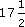These extracts are from M208 © 2006 The Open University.

All material contained within this course originated at The Open University.

Course image: Matt in Flickr made available under Creative Commons Attribution 2.0 Licence.

Don't miss out:

Author(s): The Open University

This free course provided an introduction to studying Mathematics. It took you through a series of exercises designed to develop your approach to study and learning at a distance and helped to improve your confidence as an independent learner.

Author(s): The Open University

Section 6 contains solutions to the exercises that appear throughout sections 1-5.

Click the link below to open the solutions (13 pages, 232KB).

Section 6

Author(s): The Open University

In Section 5 we show how functions may be used to sketch curves in the plane, even when these curves are not necessarily the graphs of functions.

Click the link below to open Section 5 (8 pages, 151KB).

Section 5

Author(s): The Open University

These extracts are from M208 © 2006 The Open University.

All other materials contained within this course originated at The Open University.

Except for third party materials and otherwise stated (see terms and conditions), this content is made available under a Creative Common
Author(s): The Open University

In Section 4 we introduce the hyperbolic functions sinh, cosh and tanh, which are constructed from exponential functions. These hyperbolic functions share some of the properties of the trigonometric functions but, as you will see, their graphs are very different.

Click the link below to open Section 4 (5 pages, 104KB).

Section 4
Author(s): The Open University

In Section 1 we formally define real functions and describe how they may arise when we try to solve equations. We remind you of some basic real functions and their graphs, and describe how some of the properties of these functions are featured in their graphs.

Click the link below to open Section 1 (12 pages, 1.8MB).

Section 1
Author(s): The Open University

After studying this course, you should be able to:

• understand the definition of a real function

• use the notation for intervals of the real line

• recognise and use the graphs of the basic functions described in the audio section

• understand the effect on a graph of translations, scalings, rotations and reflections

• understand how the shape of a graph of a function features properties of the function such as increasing, decr
Author(s): The Open University

Except for third party materials and otherwise stated (see terms and conditions), this content is made available under a Creative Commons Attribution-NonCommercial-ShareAlike 4.0 Licence

Course image:
Author(s): The Open UniversityStudy another free course

There are more than 800 courses on OpenLearn for you t
Author(s): The Open University

This free course provided an introduction to studying Mathematics. It took you through a series of exercises designed to develop your approach to study and learning at a distance and helped to improve your confidence as an independent learner.

Author(s): The Open University

Activity 24

A new train operator boasts ‘Train times reduced by 12%’. Decrease 90 minutes by 12%. Give your answer as minutes and seconds.

Our everyday experience of percentages includes percentage increases (like VAT at%, or a service charge of 15%) and percentage decreases (such as a discount of 15%).

For example, £8 plus
Author(s): The Open University

Fractions and decimals can also be converted to percentages, by multiplying by 100%.

So, for example, 0.17, 0.3 andcan be expressed as percentages as follows:

0.17 × 100% = 17%;

<
Author(s): The Open University

Activity 17

Express each of the following percentages as fractions:

• (a) 40%

• (b) 8%

• (c) 70%

• (d)
Author(s): The Open University

Percentages are used, particularly in newspaper articles, to indicate fractions (as in ‘64% of the population voted’) or to indicate changes (as in ‘an increase of 4%’).

Percentages often indicate proportions. For example, labels in clothes indicate the various proportions of different yarns in the fabric. ‘Per cent’ means ‘per hundred’ and is denoted by the symbol %. 100% is the same as the whole, or one hundred per hundred.

Author(s): The Open University

Activity 14

A piece of computer software is to be developed by a team of programmers. It is estimated that a team of four people would take a year. Which of the following times is the length of time taken by three program
Author(s): The Open University

In Section 2.2 you saw that direct proportion described relationships between two quantities, where as one increased, so did the other. Sometimes as one quantity increases the other decreases instead of increasing. This is called indirect proportion. Team tasks are often an example of this. The ti
Author(s): The Open University

Activity 12

A recipe for four people calls for [Image_Link]https://www.open.edu/openlearn/ocw/pluginfile.php/104648/mod_oucontent/oucontent/1902/84dcd0a9/ac42
Author(s):
The Open University

In a recipe the quantity of each ingredient needed depends upon the number of portions. As the number of portions increases, the quantity required increases. The quantity per portion is the same. This is called direct proportion. The quantity is said to be directly proportional to the number of portions. If 2 potatoes are required for one portion, 4 will be required for two portions etc. A useful method for direct proportion problems is to find the quantity for one and multiply by the
Author(s): The Open University